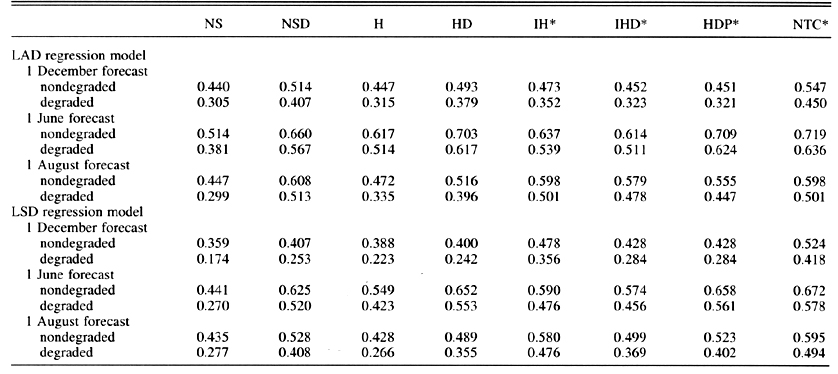Tables

Table 1a. Population consisting of 3958 noncontaminated events. Columns are (C1) true population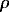values, (CS) average of 10 000 sample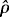values based on population regression coefficients, (C3) average of 10 000 samplevalues based on regression coefficients for each sample, and (C4) averageof 5 samplevalues for each of 10 000 random sets of regression coeficients associated with the 10 000 samples C3.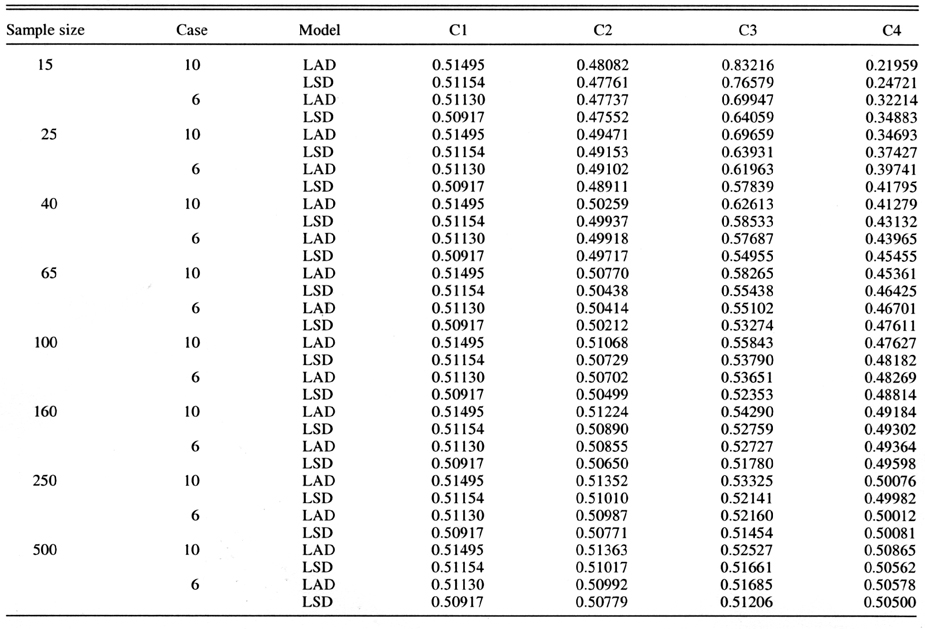Table 1b. Population 1: Initial population consisting of 3958 noncontaminated events. Columns are ratio estimators C2/C1, C3/C1, C4/C1, and C4/C3 associated with C1, C2, C3, and C4 of Table 1a.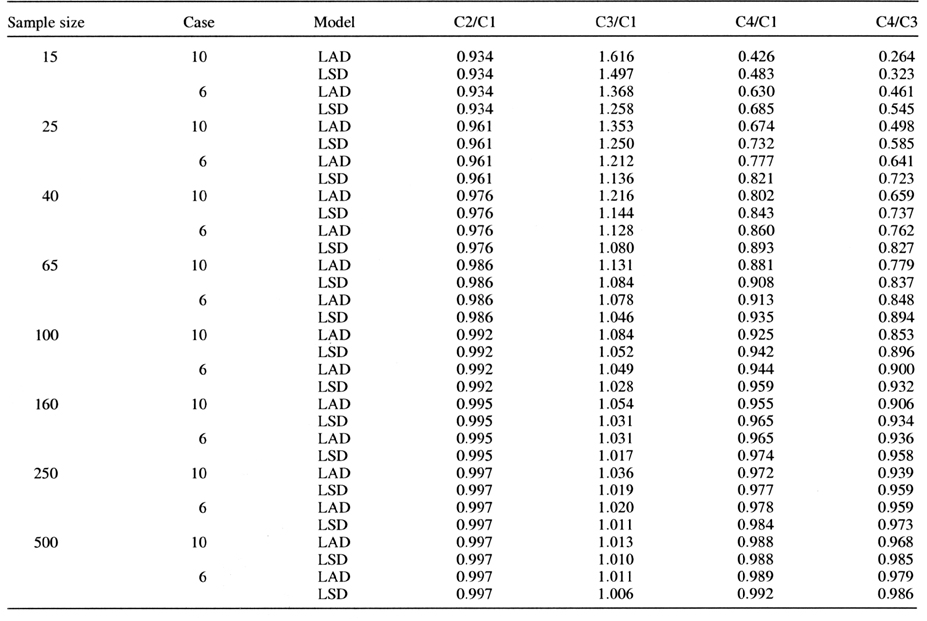Table 2a Population2: Contaminated population of 3998 events consisting of the initial population of 3958 events and 40 moderately extreme events. Columns are (C1) true populationvalues, (C2) average of 10 000 samplevalues based on population regression coefficients, (C3) average of 10 000 samplevalues based on regression coefficient for each sample, and (C4) average of 5 samplevalues for each of 10 000 random sets of regression coefficients associated with the 10 000 samples of C3.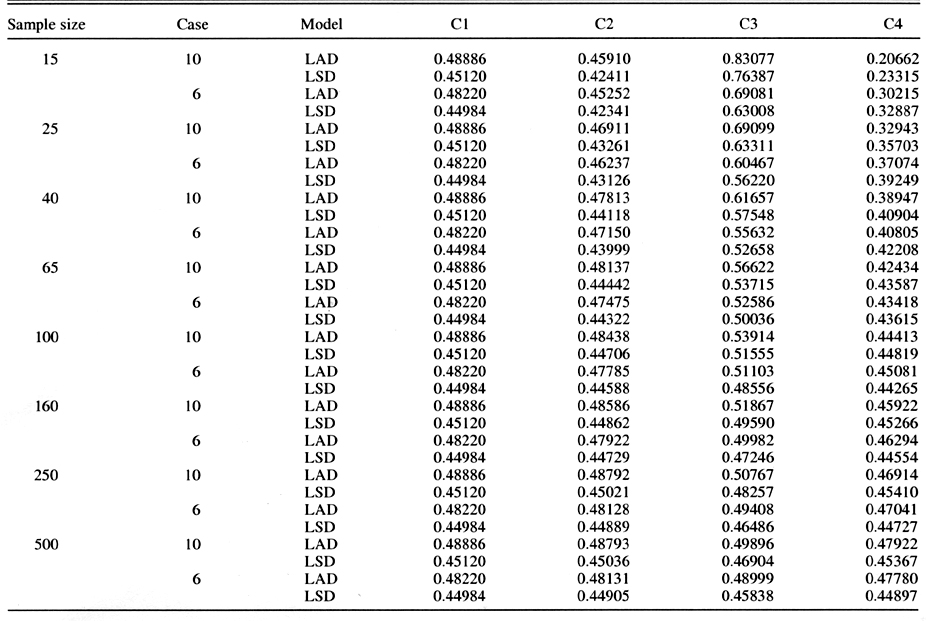Table 2b. Population 2: Contaminated population of 3998 events consisting of the initial population of 3958 events and 40 moderately extreme events. Columns are ratio estimators C2/C1, C3/C1, C4/C1, and C4/C3 associated with C1, C2, C3, and C4 of Table 2a.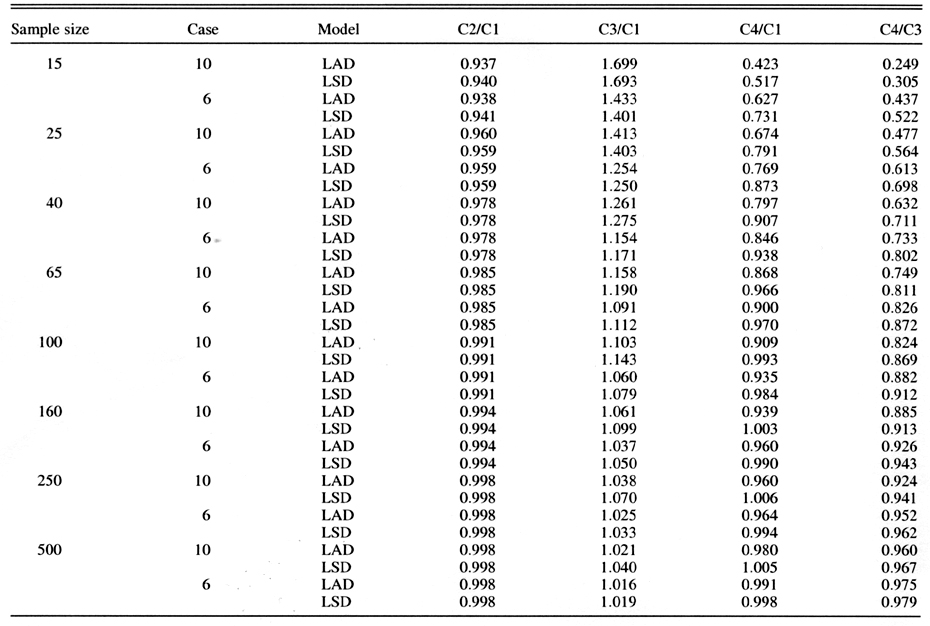Table 3a.Population of 3998 events consisting of the initial population of 3958 events and 40 very extreme events. Columns are (C1) true populationvalues, (C2) average of 10 000 samplevalues based on population regression coeficients, (C3) average of 10 000 samplevalues based on regression coefficients for each sample, and (C4) average of 5 samplevalues for each of 10 000 random sets of regression coeffcients associated with the 10 000 samples of C3.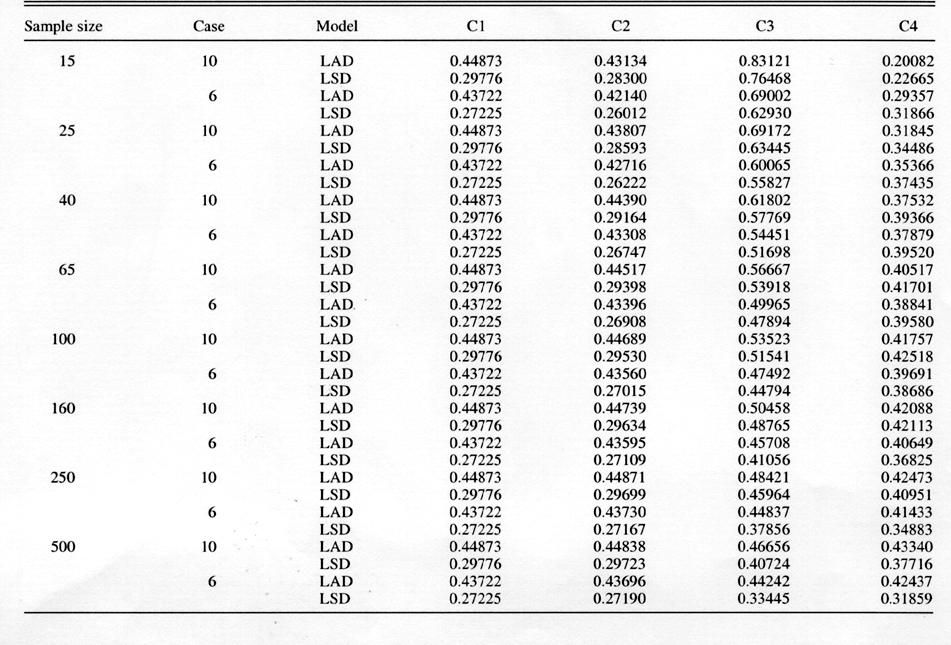Table 3b. Population 3: Contaminated population of 3998 events consisting of the initial population of 3958 events and 40 very extreme events. Columns are ratio estimators C2/C1, C3/C1, C4/C1, and C4/C3 associated with C1, C2, C3, and C4 of Table 3a.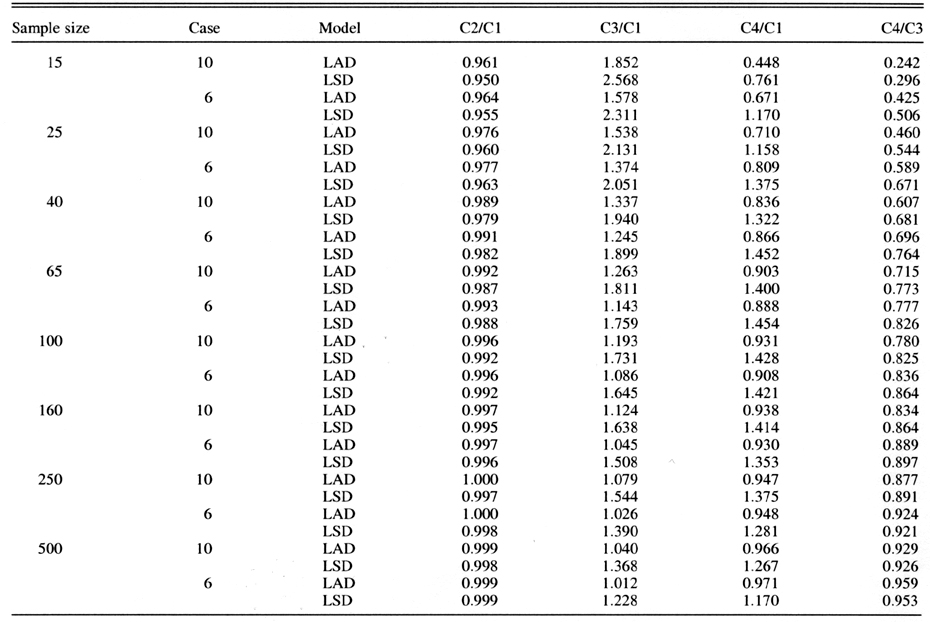Table 4a. Population 4: Contaminated population of 4158 events consisting of the initial population of 3958 events and 200 moderately extreme events. Columns are (C1) true populationvalues, (C2) average of 10 000 samplevalues based on population regression coefficients, (C3) average of 10 000 samplevalues based on regression coefficients for each sample, an (C4) average of 5 samplevalues for each of 10 000 random sets of regression coefffients associated with the 10 000 samples of C3.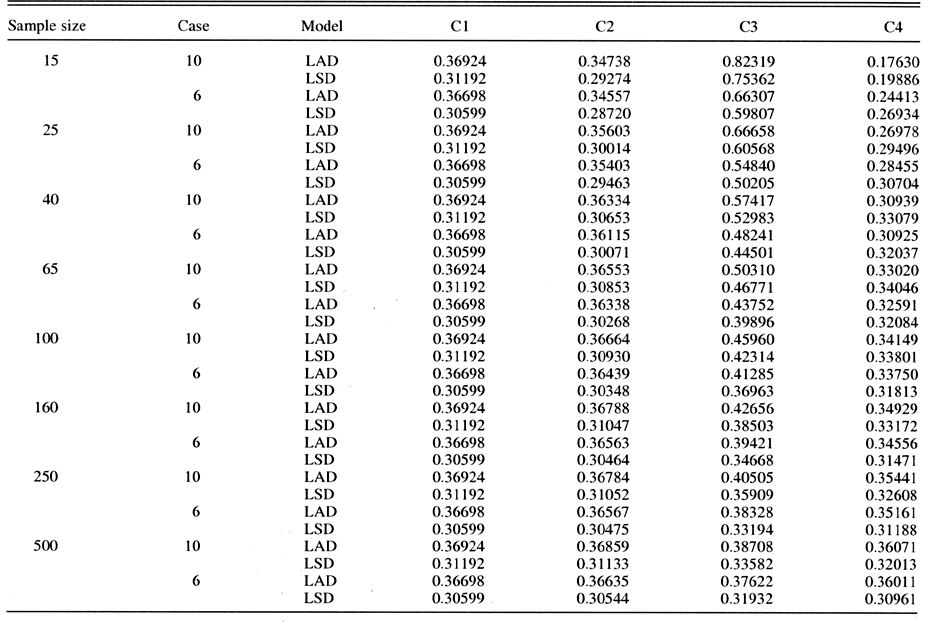Table 4b. Population 4: Contaminated population of 4158 events consisting of the initial population of 3958 events and 200 moderately exteme events. Columns are ratio estimators C2/C1, C3/C1, C4/C1, and C4/C3 associated with C1, C2, C3, and C4 of Table 4a.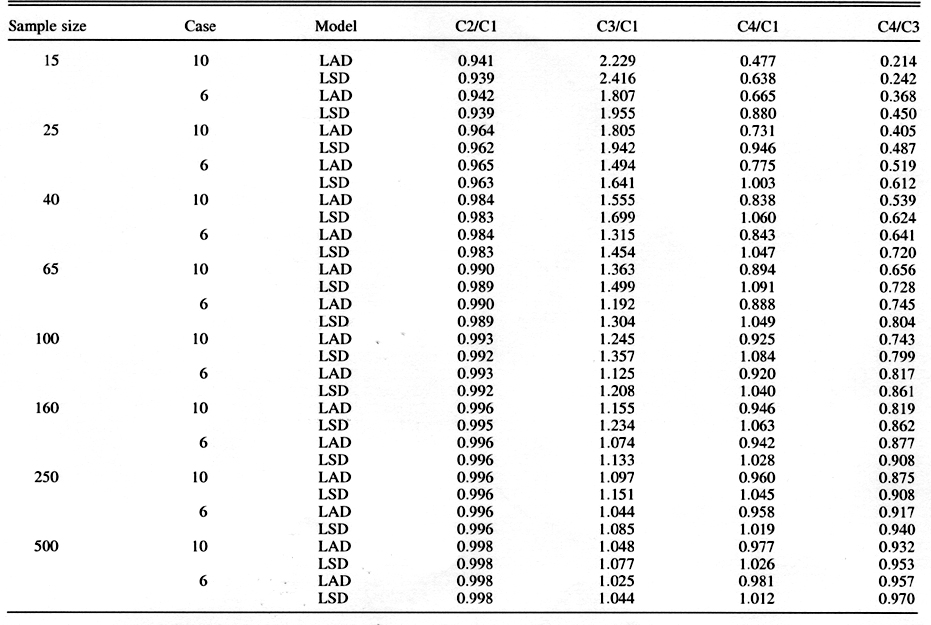Table 5a. Population 5: Contaminated population of 4158 events consisting of the initial population of 3958 events and 200 very extreme events. Columns are (C1) true populationvalues, (C2) average of 10 000 samplevalues based on population regression coefficients, (C3) average of 10 000 samplevalues based on regression coefficients for each sample, and (C4) average of 5 samplevalues for each of the 10 000 random sets of regression coefficients associated with the 10 000 samples of C3.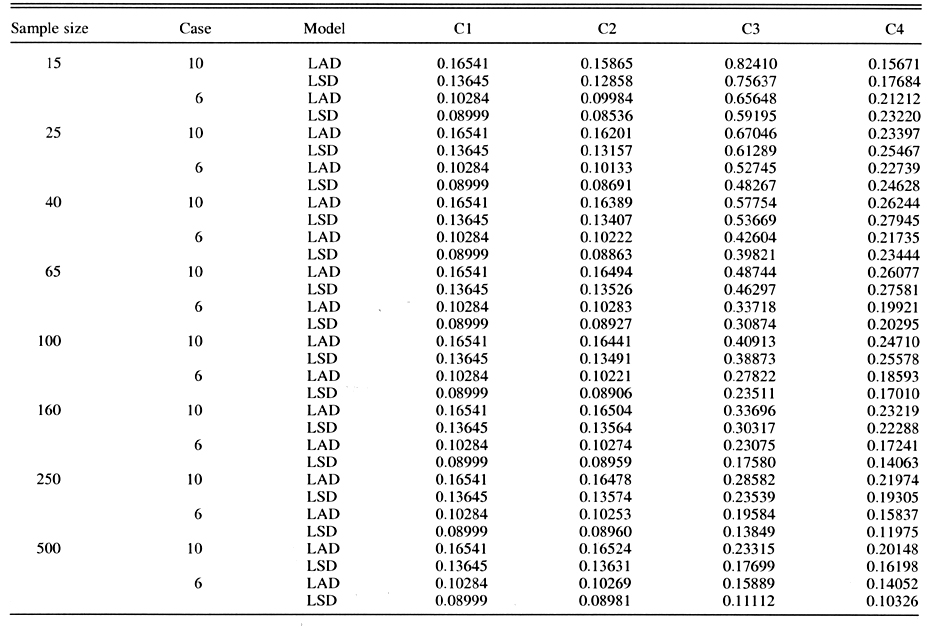Table 5b. Population 5: Contaminated population of 4158 events consisting of the initial population of 3958 events and 200 very extreme events. Columns are ratio estimators C2/C1, C3/C1, C4/C1, and C4/C3 associated with C1, C2, C3 and C4 of Table 5a.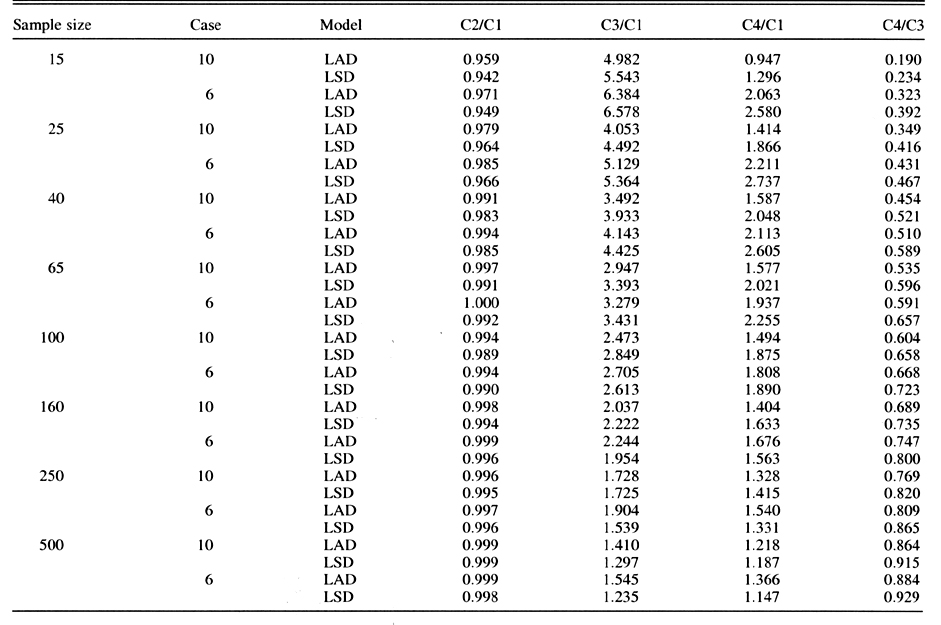Table 6. Nondegraded and degraded measures of agreement associated with LAD and LSD regression models. Column headings indicate the seasonal total of NS (named storms), H (hurricane days), IH* (intense of major hurricane), IHD* (intense hurricane days), HDP* (hurricane destruction potential), and NTC* (net tropical cyclone activity ). Note that the data with asterisks are bias corrected following the analysis of Landsea (1993).